# Crossed Polarizers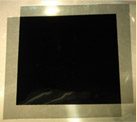An ideal polarizer produces linearly polarized light from unpolarized light. Two ideal polarizers would eliminate all light if their transmission directions are placed at right angles. The two sheets of polaroid at left are crossed and placed on an overhead projector. Polaroid is not an ideal polarizer, so some light is transmitted even when the sheets are crossed.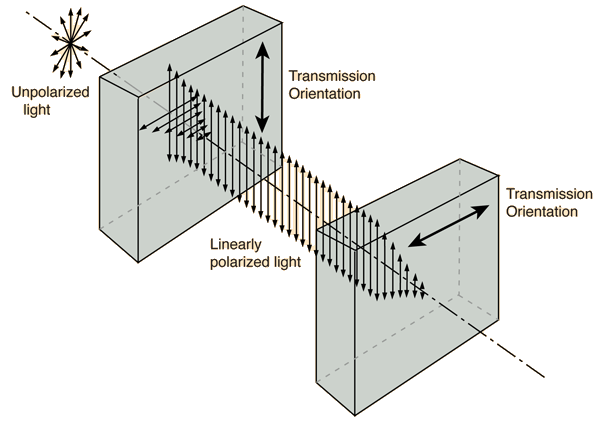Polaroid materials accomplish polarization by dichroism. At angles other than 90°, the transmitted intensity is given approximately by the Law of Malus.

 What happens if you put a third polarizer at 45° between them?
Index

Polarization concepts

 HyperPhysics***** Light and Vision R Nave
Go Back

# Polarizer Puzzle

If crossed polarizers block all light, why does putting a third polarizer at 45° between them result in some transmission of light?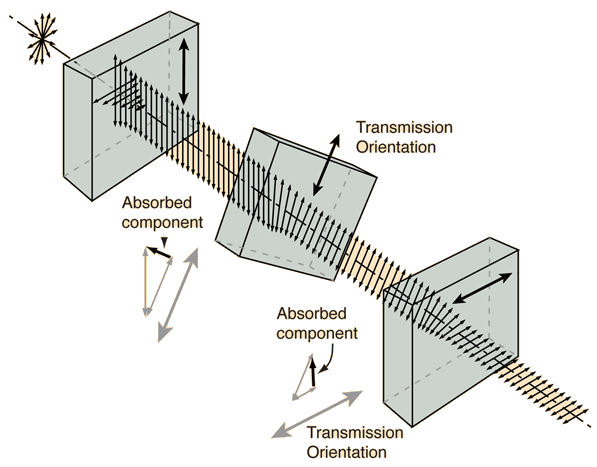In dichroic materials like polaroid, the component of the field perpendicular to the transmission plane is selectively absorbed. This achieves a rotation of the plane of polarization, but the mechanism is different from that in optically active materials.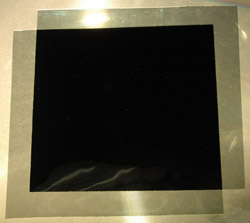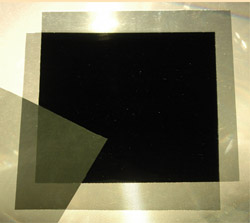In the image at left above, the polaroids are crossed, resulting in minimum transmission. At right above, a third sheet of polaroid is inserted between the crossed polarizers. In the situation shown, the transmitted intensity can be calculated by applying the Law of Malus twice. If the center polarizer is placed at 45° between crossed polarizers, 25% of the light will be transmitted.

Index

Polarization concepts

 HyperPhysics***** Light and Vision R Nave
Go Back

# Law of Malus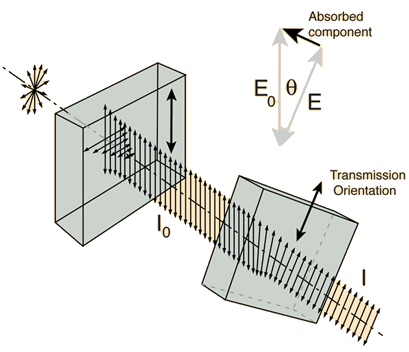When a second polarizer is rotated, the vector component perpendicular to its transmission plane is absorbed, reducing its amplitude to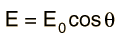Since the transmitted intensity is proportional to the amplitude squared, the intensity is given by: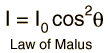If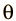= °
then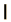=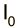The Law of Malus gives the transmitted intensity through two ideal polarizers. Note that it gives zero intensity for crossed polarizers. Real physical polarizers like sheets of polaroid are not ideal polarizers.

Index

Polarization concepts

 HyperPhysics***** Light and Vision R Nave
Go Back